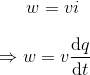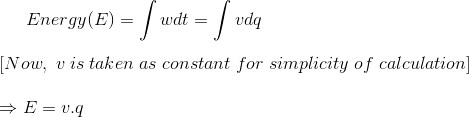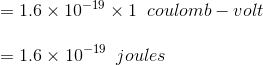# Electron volt or eV

The concept of electron volt is very simple. Let us start from very basic. We know the unit of power is watt.
W = VI, where V is the voltage and I is the current.
Now as I is current, it is nothing but rate of charge transfer. Therefore, instantaneous impression of power would beWhere, q(t) is the amount of charge transferred in time t.

Now energy is expressed asWhere, q is the charge in Coulomb crosses a voltage V volts.

From the expression of energy we can write the energy required or work to be done for crossing an electric field of total voltage V by a charge Q coulomb is QV coulomb – volt or joules. Now we know the charge of an electron is – 1.6 × 10-19 coulomb and consider it has crossed an electric field of total voltage 1 V. Then the total work to be done is charge of electron × 1 V.This amount of energy is considered as a micro unit of energy called electron-volt.

## Definition of Electron – volt

One electron – volt is the unit of energy in joules which equals to the amount of work to be done for bringing one electron against an electric field of potential difference 1 volt.
This is very tiny or micro unit of energy mainly used for different calculation in atomic and electronic levels. The concept of energy levels in the materials is dealt with this micro unit of energy that is electron volt. Not only the energy of electrons, this unit is also used for all types of energy like thermal, light etc.

### Video Presentation on Electron volt or eV

Want To Learn Faster? 🎓
Get electrical articles delivered to your inbox every week.
No credit card required—it’s 100% free.